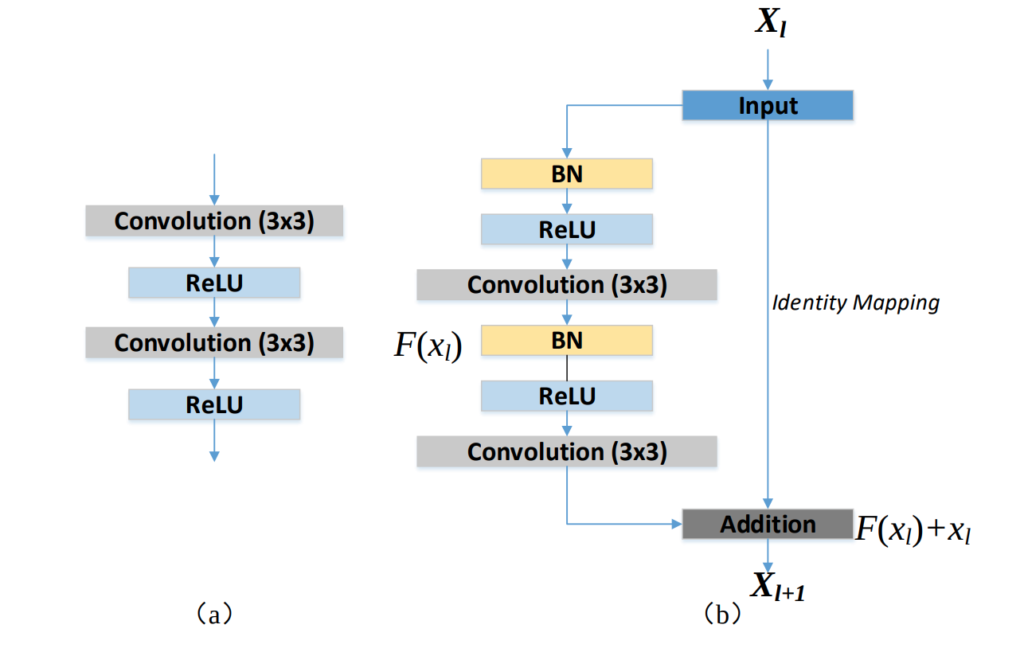# RESUNET Implementation in PyTorch

This tutorial focuses on implementing the image segmentation architecture called Deep Residual UNET (RESUNET) in the PyTorch framework. It’s an encoder-decoder architecture developed by Zhengxin Zhang et al. for semantic segmentation. It was initially used for road extraction from high-resolution aerial images in the field of remote sensing image analysis.

Original Paper: Road Extraction by Deep Residual U-Net

## RESUNET – Network Architecture

The Deep Residual Network or the RESUNET is an improvement over the existing UNET architecture by replacing the convolution block used in UNET with the residual block with identity mapping. We can say that RESUNET takes advantage of both the residual learning and the UNET architecture.(a) Block diagram of the convolution block used in UNET and (b) residual block with identity mapping used in the proposed RESUNET.

## Why Residual Learning?

Increasing the number of layers in a neural network would increase its performance. However, after a certain limit, the performance starts to decrease due to the degradation problem. He et al. proposed the residual neural network to address the degradation problem.

The residual block consists of a series of convolutional layers along with a shortcut (identity mapping) connecting both the input and the output of the block. This identity mapping helps in better flow of information, i.e., directly from one layer to another, bypassing the convolutional layers. This bypassing helps in better flow of gradients during the backpropagation.

• The use of residual blocks helps in building a deeper network without worrying about the problem of vanishing gradient or exploding gradients. It also helps in easy training of the network
• The rich skip connections in the RESUNET helps in the better flow of information between different layers, which helps in the better flow of gradients while training  (backpropagation).

## Import Torch

``````import torch
import torch.nn as nn``````

## Batch Normalization & ReLU

As the RESUNET uses a repeated application of batch normalization and then RELU. We would create a class with the name batchnorm_relu.

``````class batchnorm_relu(nn.Module):
def __init__(self, in_c):
super().__init__()

self.bn = nn.BatchNorm2d(in_c)
self.relu = nn.ReLU()

def forward(self, inputs):
x = self.bn(inputs)
x = self.relu(x)
return x``````

## Residual Block

The residual_block acts as the main block used in the entire architecture. It takes the previous feature map as input and produces an output. This output also acts as the skip connection for the decoder block.

To reduce the spatial dimensions i.e., height and width of the feature map, strided convolution is used with a stride value of 2.

``````class residual_block(nn.Module):
def __init__(self, in_c, out_c, stride=1):
super().__init__()

""" Convolutional layer """
self.b1 = batchnorm_relu(in_c)
self.c1 = nn.Conv2d(in_c, out_c, kernel_size=3, padding=1, stride=stride)
self.b2 = batchnorm_relu(out_c)
self.c2 = nn.Conv2d(out_c, out_c, kernel_size=3, padding=1, stride=1)

""" Shortcut Connection (Identity Mapping) """
self.s = nn.Conv2d(in_c, out_c, kernel_size=1, padding=0, stride=stride)

def forward(self, inputs):
x = self.b1(inputs)
x = self.c1(x)
x = self.b2(x)
x = self.c2(x)
s = self.s(inputs)

skip = x + s
return skip``````

## Decoder Block

The decoder_block takes the previous input feature map along with the skip connection from the encoder and produces and output.

``````class decoder_block(nn.Module):
def __init__(self, in_c, out_c):
super().__init__()

self.upsample = nn.Upsample(scale_factor=2, mode="bilinear", align_corners=True)
self.r = residual_block(in_c+out_c, out_c)

def forward(self, inputs, skip):
x = self.upsample(inputs)
x = torch.cat([x, skip], axis=1)
x = self.r(x)
return x``````

## RESUNET Architecture

``````class build_resunet(nn.Module):
def __init__(self):
super().__init__()

""" Encoder 1 """
self.c11 = nn.Conv2d(3, 64, kernel_size=3, padding=1)
self.br1 = batchnorm_relu(64)
self.c12 = nn.Conv2d(64, 64, kernel_size=3, padding=1)
self.c13 = nn.Conv2d(3, 64, kernel_size=1, padding=0)

""" Encoder 2 and 3 """
self.r2 = residual_block(64, 128, stride=2)
self.r3 = residual_block(128, 256, stride=2)

""" Bridge """
self.r4 = residual_block(256, 512, stride=2)

""" Decoder """
self.d1 = decoder_block(512, 256)
self.d2 = decoder_block(256, 128)
self.d3 = decoder_block(128, 64)

""" Output """
self.output = nn.Conv2d(64, 1, kernel_size=1, padding=0)
self.sigmoid = nn.Sigmoid()

def forward(self, inputs):
""" Encoder 1 """
x = self.c11(inputs)
x = self.br1(x)
x = self.c12(x)
s = self.c13(inputs)
skip1 = x + s

""" Encoder 2 and 3 """
skip2 = self.r2(skip1)
skip3 = self.r3(skip2)

""" Bridge """
b = self.r4(skip3)

""" Decoder """
d1 = self.d1(b, skip3)
d2 = self.d2(d1, skip2)
d3 = self.d3(d2, skip1)

""" output """
output = self.output(d3)
output = self.sigmoid(output)

return output``````

The code above the complete implementation of the RESUNET architecture in the PyTorch framework.1.Michal says: